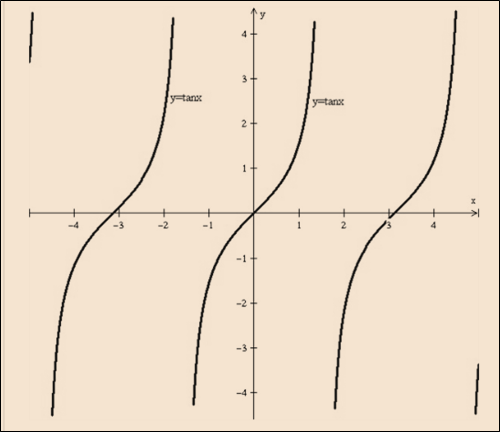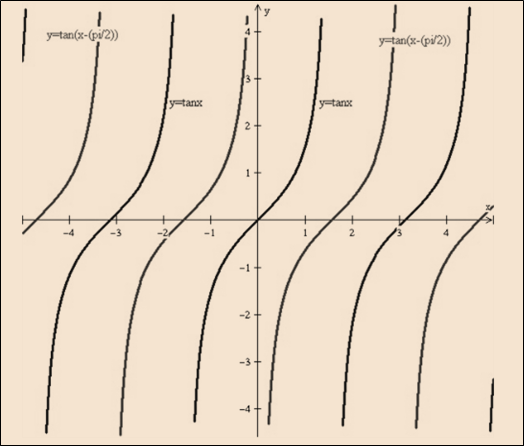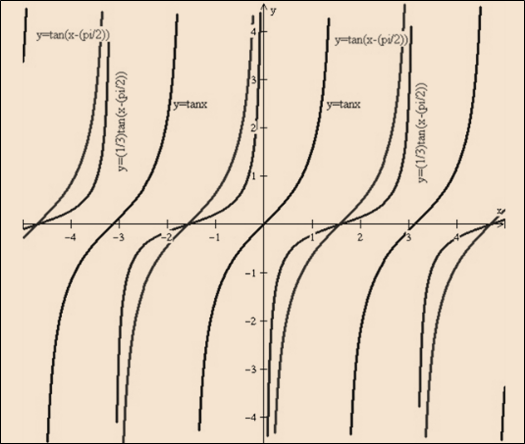# To graph: The following function with the help of the transformation: y = 1 3 tan ( x − π 2 )### Single Variable Calculus: Concepts...

4th Edition
James Stewart
Publisher: Cengage Learning
ISBN: 9781337687805### Single Variable Calculus: Concepts...

4th Edition
James Stewart
Publisher: Cengage Learning
ISBN: 9781337687805

#### Solutions

Chapter C, Problem 39E
To determine

## To graph: The following function with the help of the transformation:   y=13tan(x−π2)

Expert Solution

### Explanation of Solution

Given information:

y=13tan(xπ2)

Graph:Graph 1: y=tanxGraph 2: y=tan(xπ2)Graph 3: y=13tan(xπ2)

Interpretation:

Need to draw a first graph of the function y=tanx as shown by the graph 1. Now, the given function y=13tan(xπ2) is in form of the y=f(xc) . It means that need to shift the graph by y=f(x)c on the right side.

Hence, the graph is shifted towards right y=tanx by (π2) it shown by the graph 2. Shrink the graph y=f(x) by factor c , write the function in the form of y=1cf(x) .

Hence, by factor 3 , the graph of y=tan(xπ2) shrink shown by the graph 3 of y=tan(xπ2) .

### Have a homework question?

Subscribe to bartleby learn! Ask subject matter experts 30 homework questions each month. Plus, you’ll have access to millions of step-by-step textbook answers!